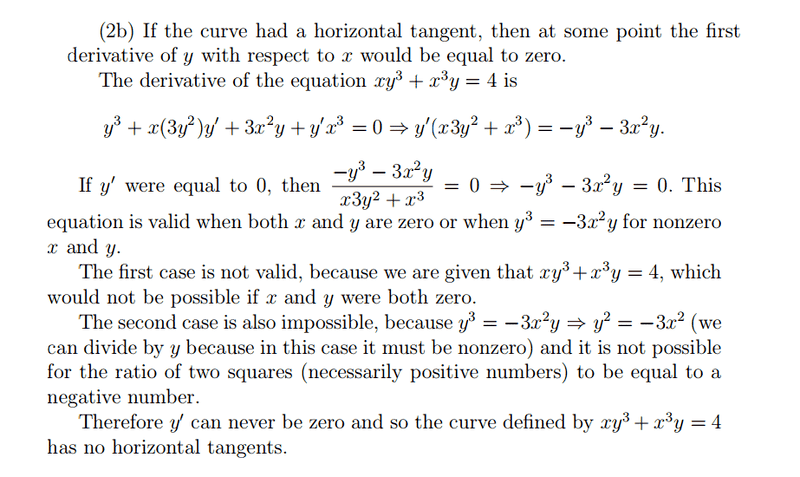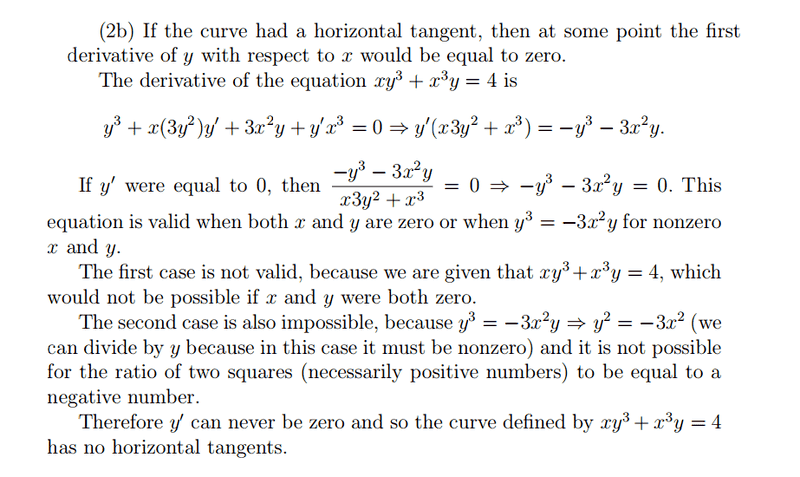# Can someone explain this answer "in layman's terms"?

• iwantcalculus

#### iwantcalculus

The question is :

Show that the curve defined implicitly by the equation

X*Y^3 + x^3*Y = 4

has no horizontal tangent.Can someone please explain what steps are used in order to obtain the answer in a simple way for a calculus beginner? This answer is not helping me understand the question and how to answer the question ( It is too complicated and gets me confused).

And if you have similar questions, please show me them so I can practice on them after I understand this question in order to be comfortable with this type of questions.

A curve is given ##f(x,y) = c ##. To see if there are any horizontal tangents, you need to get ##y' = \frac{dy}{dx} ##. So then you take the derivative of both sides of the equation ##f(x,y) = c ## resulting in some other true equation ##f'(x,y) = 0 ##. You can obtain ##f'## by implicit differentiation. Now somewhere in ##f'## there will be a ##dy/dx## aka ##y'##. You need to extract that somehow and solve for it. How you do this will depend on the the function you were given. In this case ##y'## is that that ratio of two polynomials.

To find if the function has a horizontal tangent we solve ##dy/dx = 0##. In our case ##dy/dx## is a fraction of two things $$y' = \frac{a(x,y)}{b(x,y)} = 0$$ can only be true if ##a(x,y)= 0##. So to find solutions set the numerator to zero (note however that ##a(x,y) = 0 ## and ##b(x,y) = 0## is a special case). Finally one goes on to see if there is a solution to ##dy/dx= y' = 0##. One finds in this case that there isn't but in general there might be.

Last edited:
The question is :

Show that the curve defined implicitly by the equation

X*Y^3 + x^3*Y = 4

has no horizontal tangent.The above is not "the answer." It is the explanation of why the curve xy3 + yx3 = 4 doesn't have a horizontal tangent. The explanation seems pretty straightforward to me. What part of the explanation seems confusing to you?
iwantcalculus said:
Can someone please explain what steps are used in order to obtain the answer in a simple way for a calculus beginner? This answer is not helping me understand the question and how to answer the question ( It is too complicated and gets me confused).

And if you have similar questions, please show me them so I can practice on them after I understand this question in order to be comfortable with this type of questions.

Perhaps a little bit simpler: a "horizontal tangent" is where y'= dy/dx= 0. Differentiating both sides of $xy^3+ x^3y= 4$ with respect to x,
$y^3+ 3xy^2y'+ 3x^2y+ x^3y'= 0$.

At any point where y'= 0, that becomes $y^3+ 3x^2y= y(y^2+ 3x^2)= 0$. That is only true where y= 0 or $y^2+ 3x^2= 0$ which, again, requires that y= 0. But if y= 0 then $xy^3+ x^2y= 0$, not 4. That curves does not contain any point where y= 0 so no point where y'= 0.

I think that the original explanation is pretty good and thorough, step-by-step. It uses a lot of fundamental facts. You should look at it carefully step-by-step and ask about the specific steps that are a problem.

•Mark44